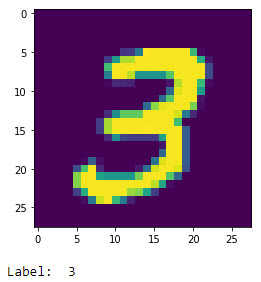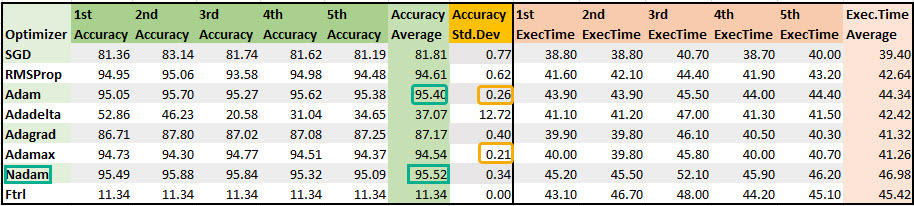# Neural Networks - Types of Optimizers and PerformancePhoto by Uriel SC on Unsplash

The goal of any machine learning algorithm is to reduce the loss. To minimize the loss, optimizing algorithms help us find the local minima at which the loss is the least.

There are different type of optimizers and the discussion regarding what precisely each does, the advantages and disadvantages of these optimizers have been discussed widely on the internet like this one here

This article takes these optimizers and places it at the next level. Finding the best optimizer for a classification dataset using the default parameters. We will see the time is taken for the code to complete and its accuracy only by using the same parameters across the optimizers.

### Dataset

We will use the MNIST handwritten digits dataset for this classification problem. MNIST database is a set of images of handwritten digits, and the goal of the deep learning model is to predict the number written correctly. It is a multi-classification problem with digits 0 to 9.

We will use Keras and the Tensorflow backend for this problem.

• Import the necessary libraries
import matplotlib.pyplot as plt
from sklearn.model_selection import train_test_split
from keras.datasets import mnist
from keras.models import Sequential
from keras.utils.np_utils import to_categorical
from keras.layers import Activation, Dense, BatchNormalization
from keras import optimizers

• Load the dataset from mnist
(X_train, y_train), (X_test, y_test) = mnist.load_data()

# 11493376/11490434 [==============================] - 7s 1us/step

• Show any number from the dataset to see if it’s working
plt.imshow(X_train)
plt.show()
print('Label: ', y_train)• Let’s reshape the data to convert 28 by 28 pixel data to 1 row by 784 pixels
# reshaping X data: (n, 28, 28) => (n, 784)
X_train = X_train.reshape((X_train.shape, -1))
X_test = X_test.reshape((X_test.shape, -1))

• Let’s split the data to train and test and convert the data to a categorical data
# use only 33% of training data to expedite the training process
X_train, _ , y_train, _ = train_test_split(X_train, y_train,
test_size = 0.67, random_state = 101)

# converting y data into categorical (one-hot encoding)
y_train = to_categorical(y_train)
y_test = to_categorical(y_test)


### Defining a model

Let’s us go ahead and define a model that has

• elu as the first activation function
• softmax as the last function before output (since this is a multi-classification problem)
• 784 as the number of inputs ( 28 by 28 pixels )
• he_normal as the initializer
• 5 layers
• 50 neurons in the first four layers
• 10 neurons in the last layer
• categorical_crossentropy as the loss function
• accuracy as the metrics
Types of optimizers

• We will use all the following optimizers and check the performance and accuracy it yeilds
• SGD
• RMSProp
• Ftrl

With the above parameters, let’s define a sequential model.

def mlp_model(activation_first, activation_last, input_shape, initializer,
neurons_layers_first, neurons_layers_last, loss, metrics,
optimizer):

model = Sequential()
model.add(Dense(neurons_layers_first, input_shape = (input_shape , ),
kernel_initializer=initializer))

model.compile(optimizer = optimizer, loss = loss, metrics = metrics)
return model


### Optimizers

Stochastic gradient descent performs a parameter update based on each training example. It performs one update at a time. It is much faster but can have a high variance.

sgd = optimizers.SGD(lr = 0.001)
model = mlp_model('sigmoid','softmax', 784 , 'he_normal', 50,10,
'categorical_crossentropy', ['accuracy'], sgd)

%%time
model.fit(X_train, y_train, batch_size = 256, validation_split = 0.3,
epochs = 100, verbose = 0)

# CPU times: user 37.5 s, sys: 3.17 s, total: 40.7 s
# Wall time: 26.6 s
#<tensorflow.python.keras.callbacks.History at 0x7f8a2c3d29e8>

results = model.evaluate(X_test, y_test)
print('Test accuracy: ', results*100)

# 313/313 [==============================] - 0s 899us/step
# - loss: 1.2138 - accuracy: 0.8175
# Test accuracy:  81.74999952316284


#### RMSProp

RMSProp divides the learning rate by an exponentially decaying average of squared gradients.

rms = optimizers.RMSprop(lr = 0.001)
model = mlp_model('sigmoid','softmax', 784 , 'he_normal', 50,10,
'categorical_crossentropy', ['accuracy'], rms)

%%time
model.fit(X_train, y_train, batch_size = 256, validation_split = 0.3,
epochs = 100, verbose = 0)

# CPU times: user 40.8 s, sys: 3.58 s, total: 44.4 s
# Wall time: 28.9 s
# <tensorflow.python.keras.callbacks.History at 0x7f8a2b03e198>

results = model.evaluate(X_test, y_test)
print('Test accuracy: ', results*100)

# 313/313 [==============================] - 0s 921us/step
# - loss: 0.3434 - accuracy: 0.9358
# Test accuracy:  93.58000159263611


It stores both the exponentially decaying average of past gradients and a decaying average of past squared gradients while computing the learning rate.

Adam optimizer gave the second-best accuracy for this multi-classification problem.

adam = optimizers.Adam(lr = 0.001)
model = mlp_model('sigmoid','softmax', 784 , 'he_normal', 50,10,

%%time
model.fit(X_train, y_train, batch_size = 256, validation_split = 0.3,
epochs = 100, verbose = 0)

# CPU times: user 41.3 s, sys: 4.21 s, total: 45.5 s
# Wall time: 28.9 s
# <tensorflow.python.keras.callbacks.History at 0x7f8a2ac92940>

results = model.evaluate(X_test, y_test)
print('Test accuracy: ', results*100)

# 313/313 [==============================] - 0s 925us/step
# - loss: 0.1914 - accuracy: 0.9527
# Test accuracy:  95.27000188827515


Adagrad is the algorithm that adaptively scales the learning rate for each of the dimensions. How it works is documented here

adagrad = optimizers.Adagrad(lr = 0.001)
model = mlp_model('sigmoid','softmax', 784 , 'he_normal', 50,10,

%%time
model.fit(X_train, y_train, batch_size = 256, validation_split = 0.3,
epochs = 100, verbose = 0)

# CPU times: user 41.5 s, sys: 4.6 s, total: 46.1 s
# Wall time: 30.1 s
# <tensorflow.python.keras.callbacks.History at 0x7f8a27444b00>

results = model.evaluate(X_test, y_test)
print('Test accuracy: ', results*100)

# 313/313 [==============================] - 0s 968us/step
# - loss: 1.0017 - accuracy: 0.8703
# Test accuracy:  87.02999949455261


adadelta = optimizers.Adadelta(lr = 0.001)
model = mlp_model('sigmoid','softmax', 784 , 'he_normal', 50,10,

%%time
model.fit(X_train, y_train, batch_size = 256, validation_split = 0.3,
epochs = 100, verbose = 0)

# CPU times: user 42.4 s, sys: 4.61 s, total: 47 s
# Wall time: 30.7 s
# <tensorflow.python.keras.callbacks.History at 0x7f8a28807da0>

results = model.evaluate(X_test, y_test)
print('Test accuracy: ', results*100)

# 313/313 [==============================] - 0s 945us/step
# - loss: 1.9577 - accuracy: 0.2059
# Test accuracy:  20.589999854564667


Adamax is the generalization of the Adam algorithm. It is based on the adaptive estimates of lower-order moments.

adamax = optimizers.Adamax(lr = 0.001)
model = mlp_model('sigmoid','softmax', 784 , 'he_normal', 50,10,

%%time
model.fit(X_train, y_train, batch_size = 256, validation_split = 0.3,
epochs = 100, verbose = 0)

# CPU times: user 41.4 s, sys: 4.43 s, total: 45.8 s
# Wall time: 30 s
# <tensorflow.python.keras.callbacks.History at 0x7f8a2712a048>

results = model.evaluate(X_test, y_test)
print('Test accuracy: ', results*100)

# 313/313 [==============================] - 0s 925us/step
# - loss: 0.1897 - accuracy: 0.9478
# Test accuracy:  94.77999806404114


Nadam is Adam incorporated with Nesterov momentum. Nesterov momentum is an improved version of the momentum algorithm where the momentum vector is applied to the parameters before computing the gradient.

Nadam optimizer gave the best accuracy for this dataset.

nadam = optimizers.Nadam(lr = 0.001)
model = mlp_model('sigmoid','softmax', 784 , 'he_normal', 50,10,

%%time
model.fit(X_train, y_train, batch_size = 256, validation_split = 0.3,
epochs = 100, verbose = 0)

# CPU times: user 46.9 s, sys: 5.26 s, total: 52.1 s
# Wall time: 33.1 s
# <tensorflow.python.keras.callbacks.History at 0x7f8a24cb34e0>

results = model.evaluate(X_test, y_test)
print('Test accuracy: ', results*100)

# 313/313 [==============================] - 0s 905us/step
# - loss: 0.1711 - accuracy: 0.9584
# Test accuracy:  95.8400011062622


#### Ftrl

This optimizer uses the Ftrl algorithm (Follow The Regularized Leader). Out of all the optimizers, Ftrl gave the least accuracy for this multi-classification problem.

The reason being this algorithm is designed to work when there is an interaction among the features. However, for benchmarking purposes, it is used here.

ftrl = optimizers.Ftrl(lr = 0.001)
model = mlp_model('sigmoid','softmax', 784 , 'he_normal', 50,10,
'categorical_crossentropy', ['accuracy'], ftrl)

%%time
model.fit(X_train, y_train, batch_size = 256, validation_split = 0.3,
epochs = 100, verbose = 0)

# CPU times: user 43.5 s, sys: 4.48 s, total: 48 s
# Wall time: 30.9 s
# <tensorflow.python.keras.callbacks.History at 0x7f8a23790320>

results = model.evaluate(X_test, y_test)
print('Test accuracy: ', results*100)

# 313/313 [==============================] - 0s 905us/step
# - loss: 2.3012 - accuracy: 0.1135
# Test accuracy:  11.349999904632568


### Conclusion

In using all the optimizers for this multi-classification dataset, Adam and its derivatives like Nadam and Adamax gave the best possible accuracy.

The accuracy and the execution time based on five rounds of execution on a google colab instance (without GPU runtime). Results are documented below.

Nadam gave the best accuracy with the least standard deviation but, tool a bit longer for execution